# RS Aggarwal Solutions for Class 10 Maths Chapter 1 Real Numbers Exercise 1D Page number 33

RS Aggarwal Solutions for Class 10 Maths Chapter 1 Real Numbers, contains solutions for all Exercise 1D questions. This exercise is based on concepts such as Irrational Numbers and some of its important properties. This set of questions and answers is prepared by BYJU’S subject experts to help students to do well in their exams. Download the RS Aggarwal Solutions of Class 10 now and practice all the questions.

### Download PDF of RS Aggarwal Solutions for Class 10 Maths Chapter 1 Real Numbers Exercise 1D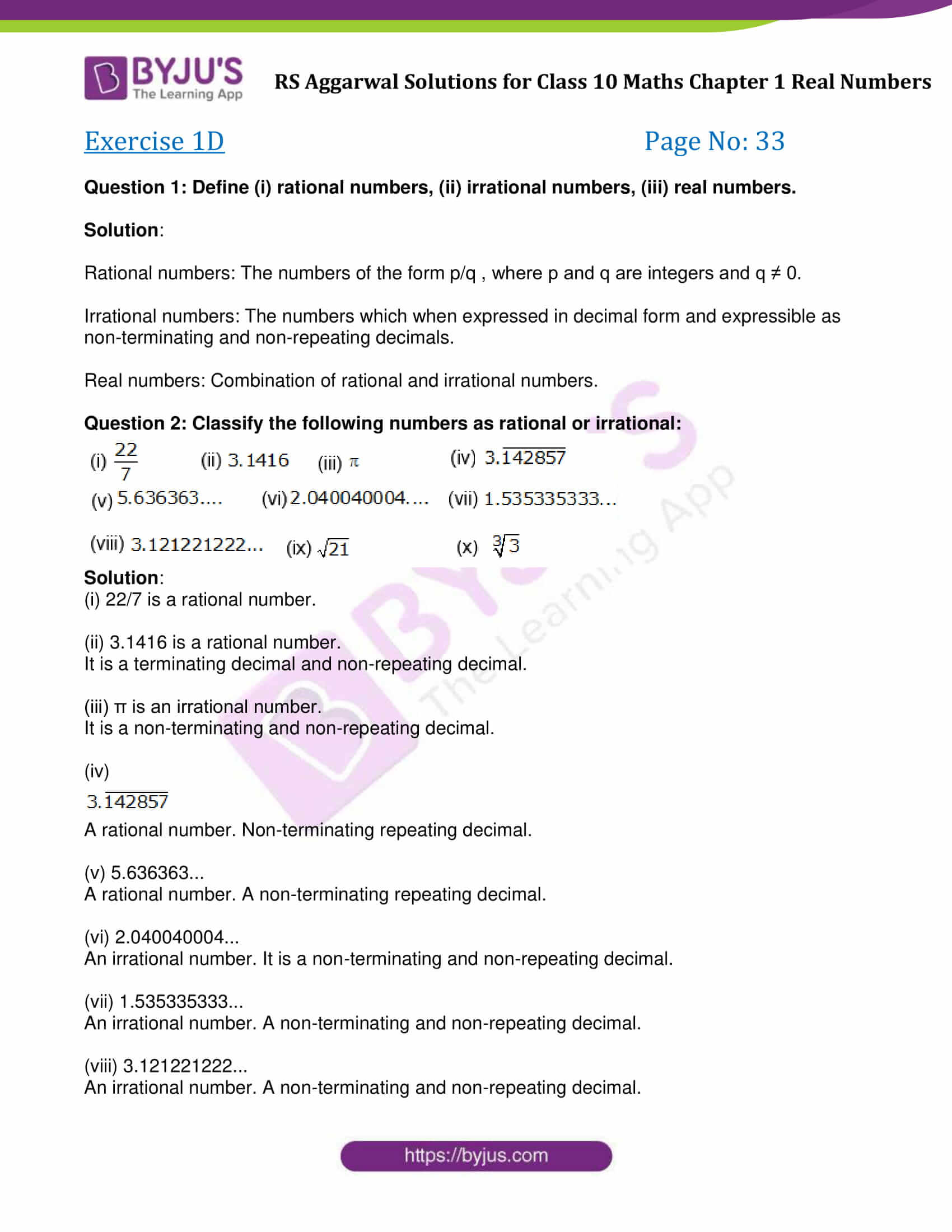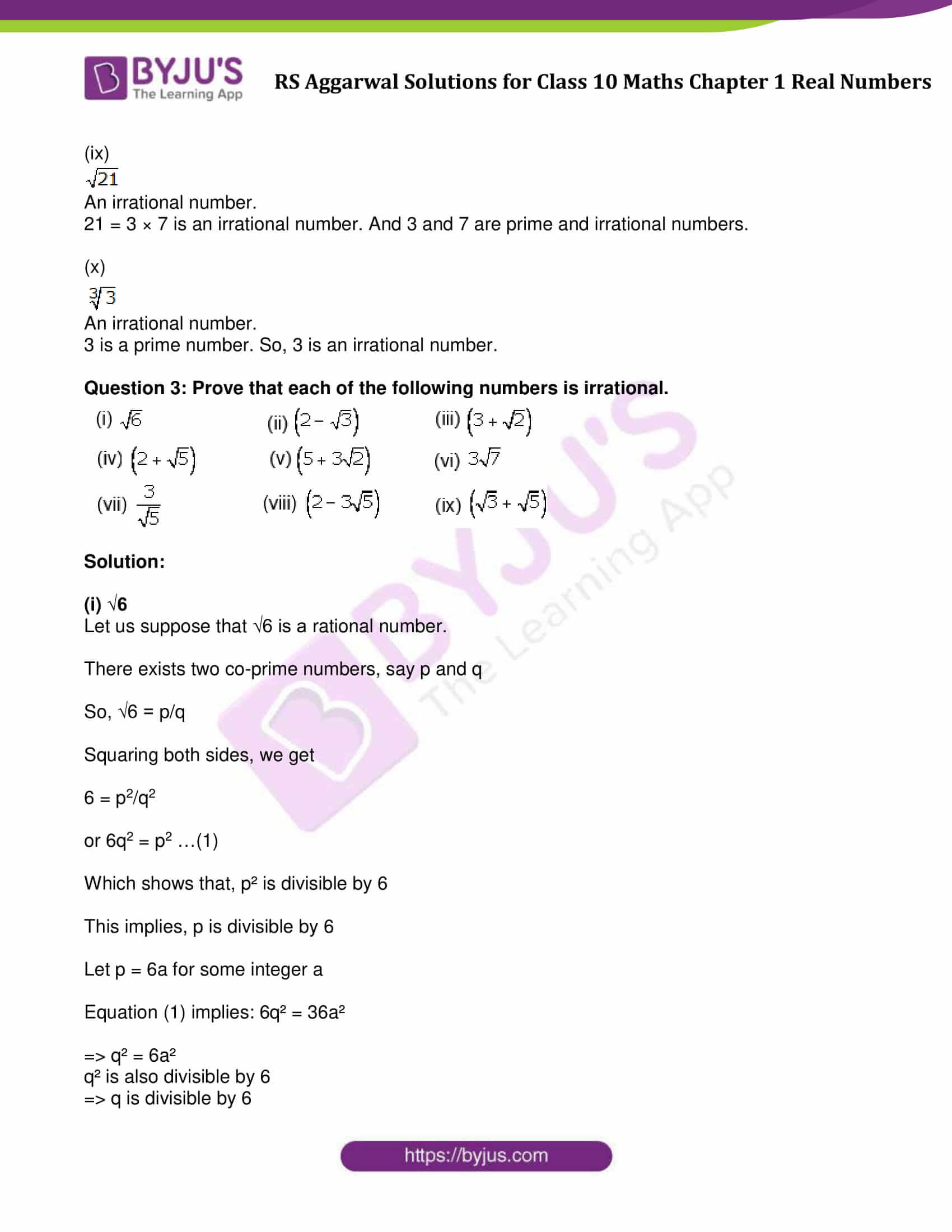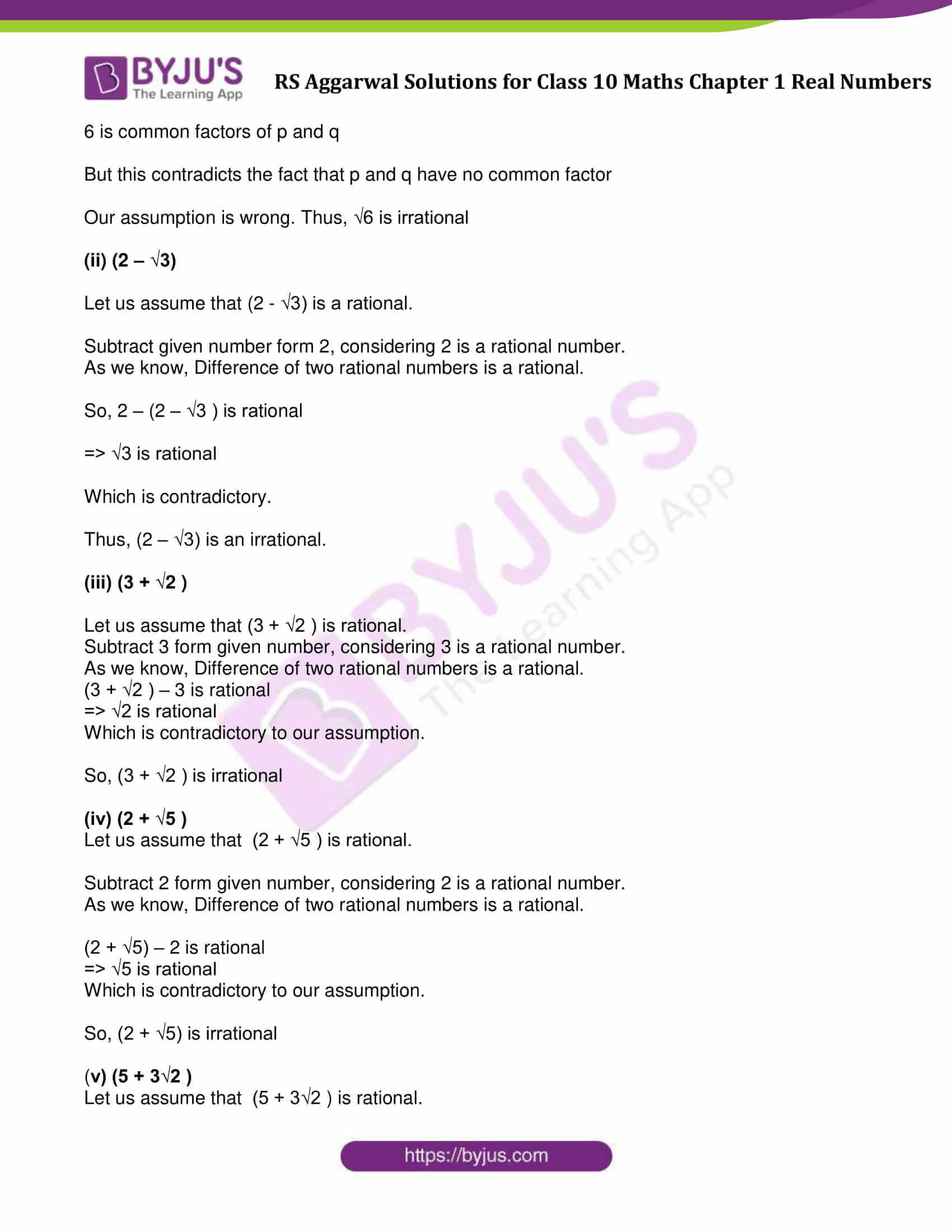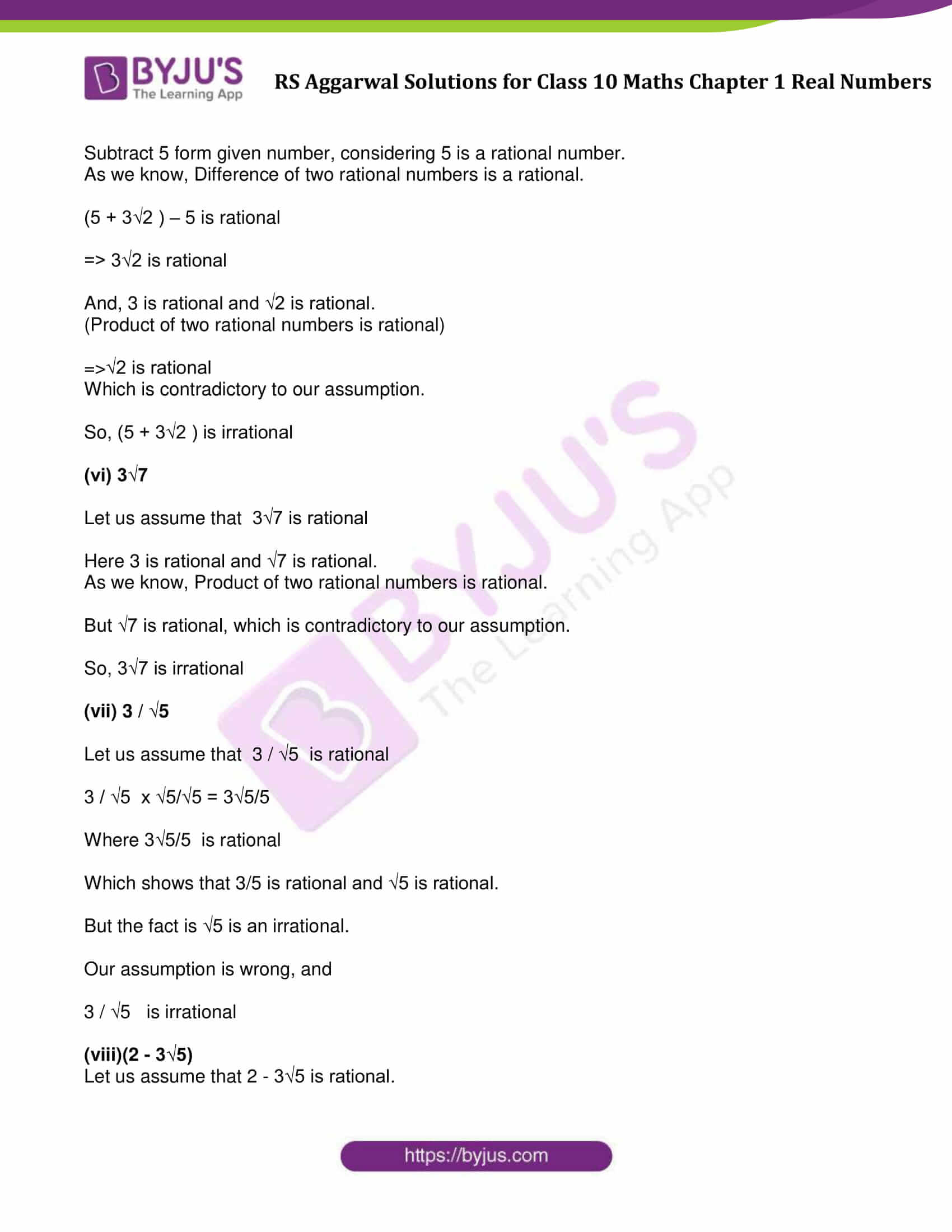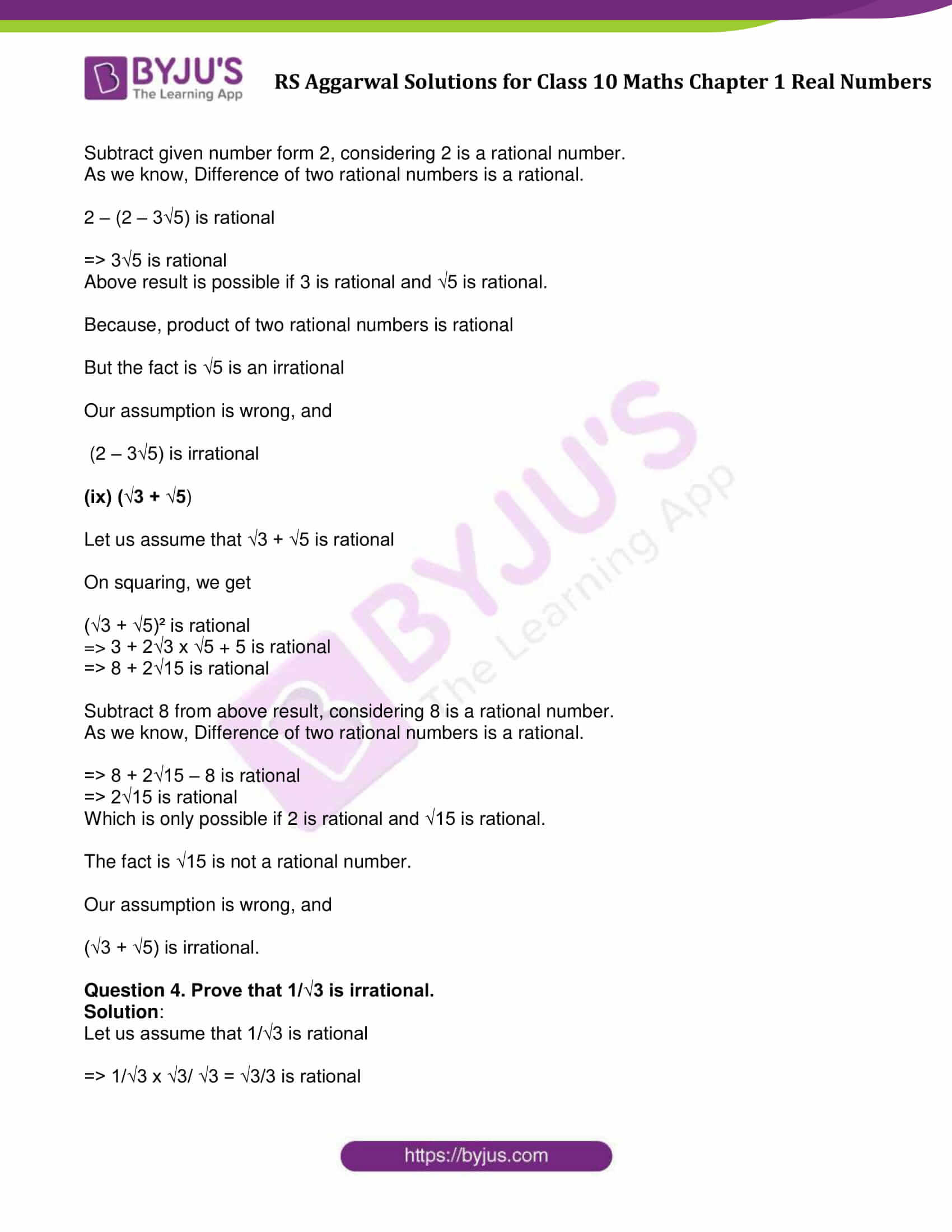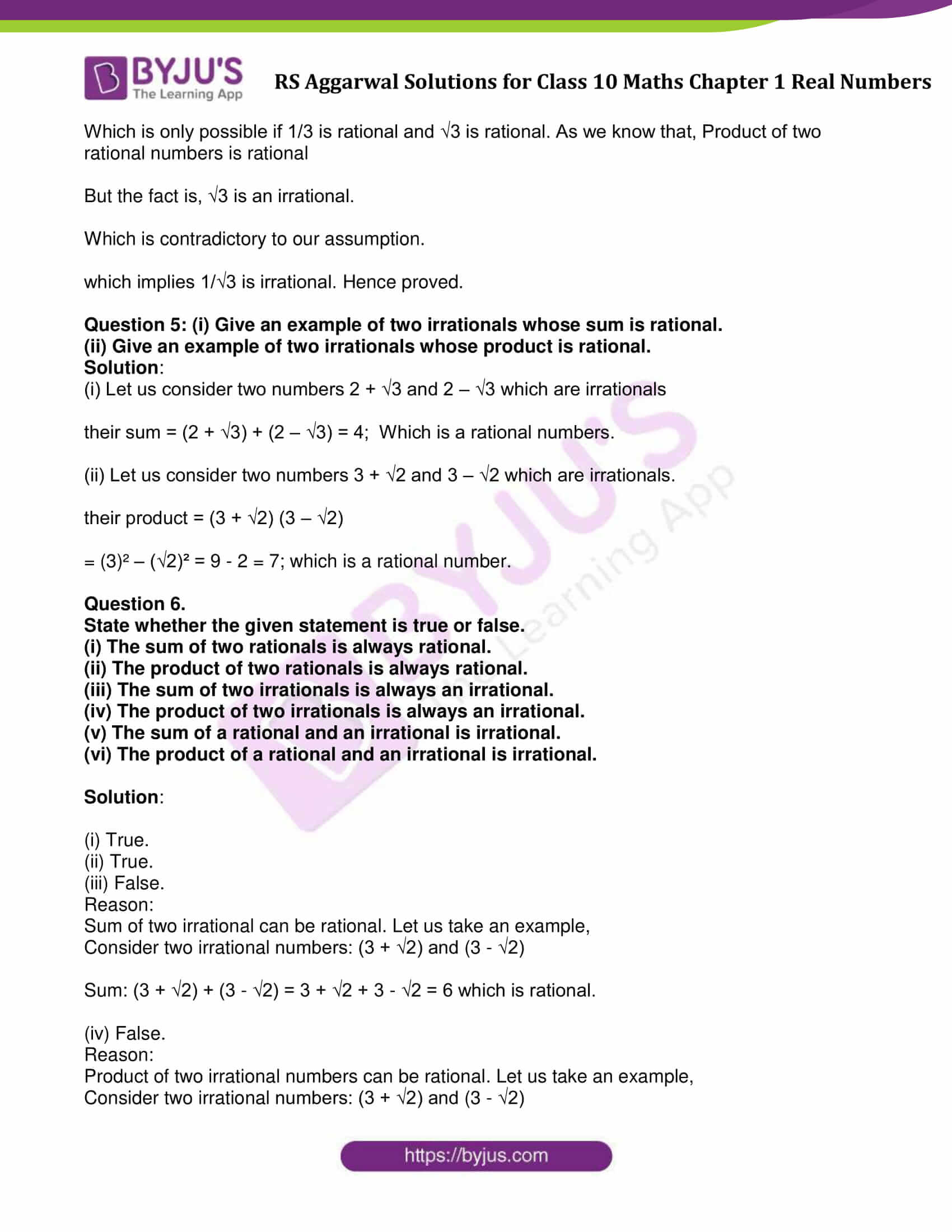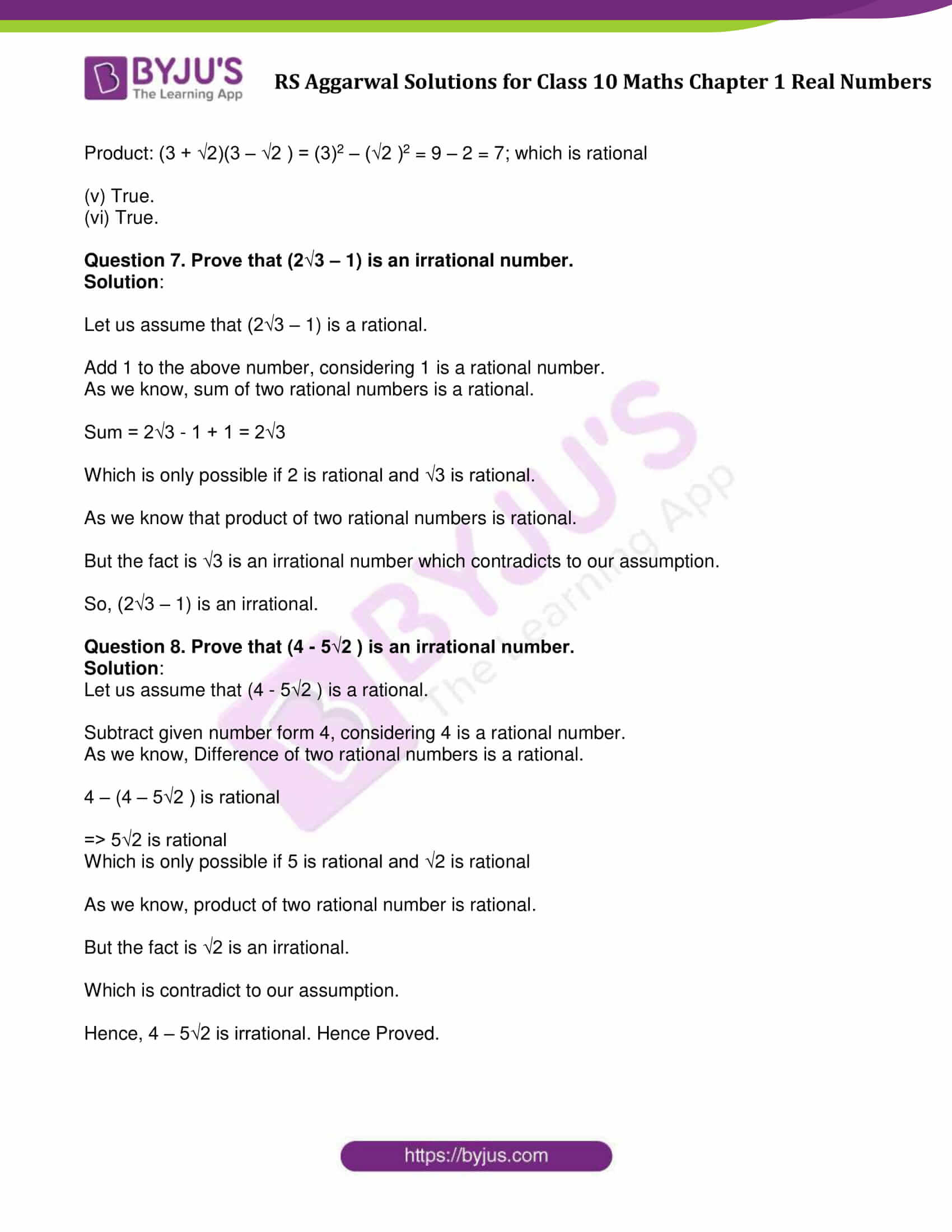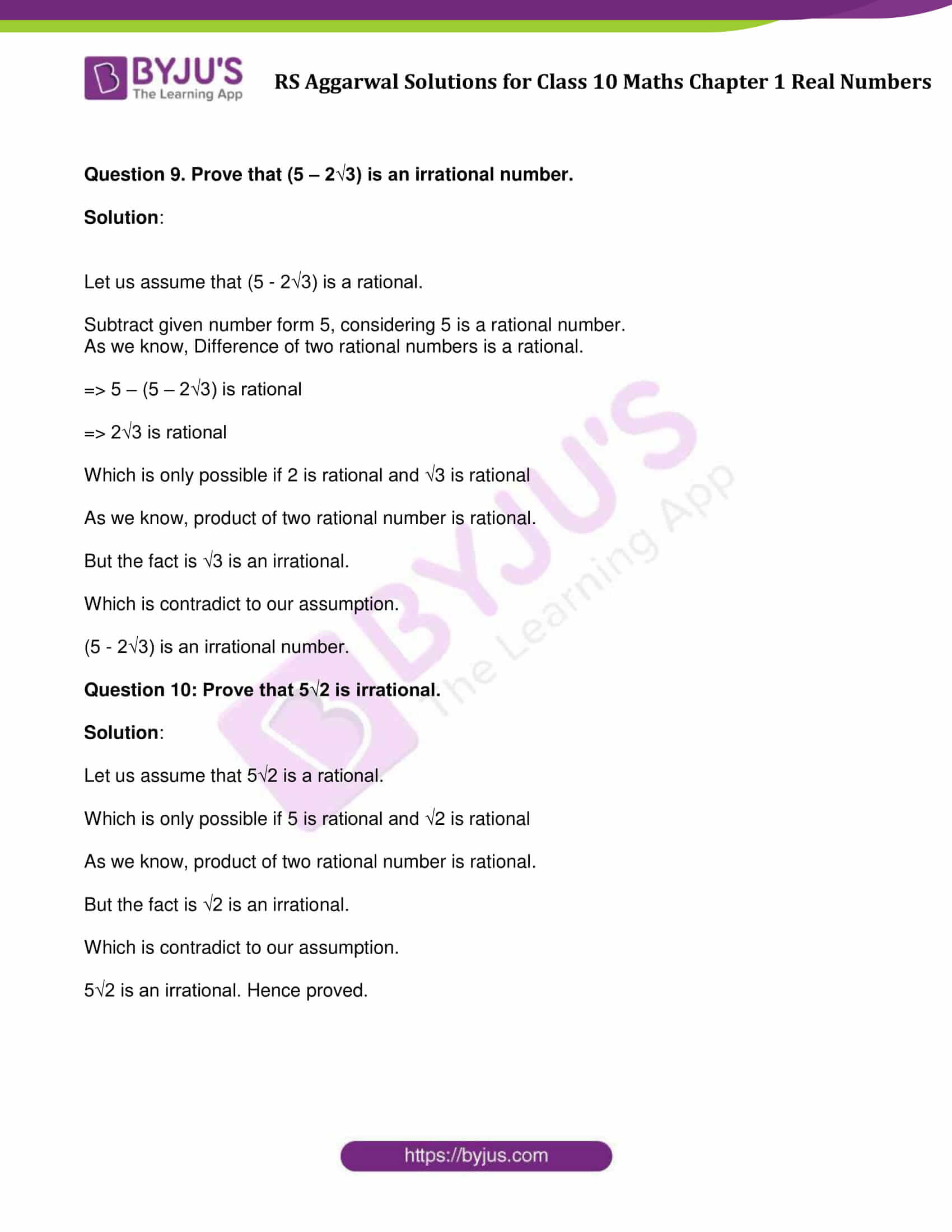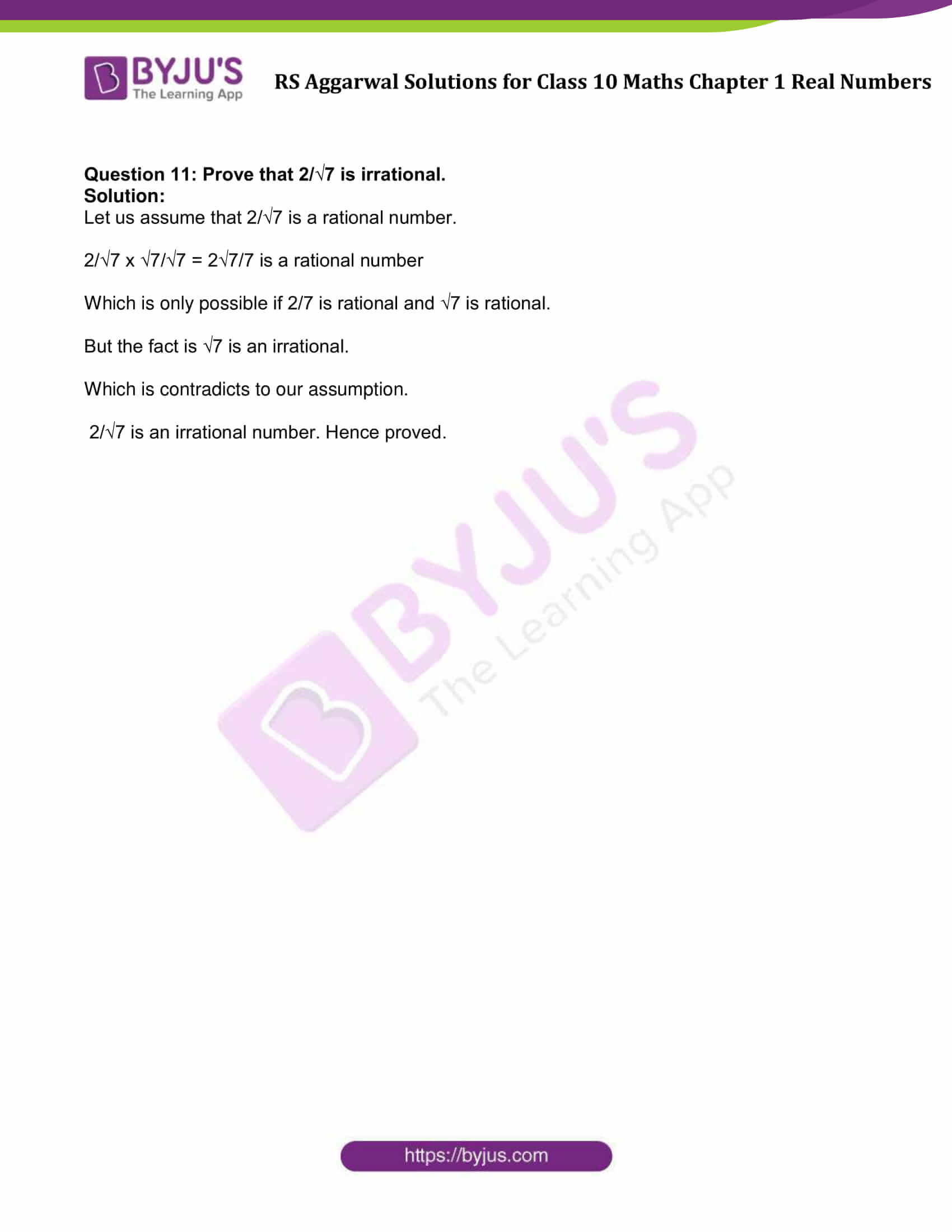### Access other exercise solutions of Class 10 Maths Chapter 1 Real Numbers

Exercise 1A Solutions : 10 Questions (Short Answers)

Exercise 1B Solutions : 27 Questions (Short Answers)

Exercise 1C Solutions : 3 Questions (Short Answers)

Exercise 1E Solutions : 23 Questions (Short Answers)

## Exercise 1D Page No: 33

Question 1: Define (i) rational numbers, (ii) irrational numbers, (iii) real numbers.

Solution:

Rational numbers: The numbers of the form p/q , where p and q are integers and q ≠ 0.

Irrational numbers: The numbers which when expressed in decimal form and expressible as non-terminating and non-repeating decimals.

Real numbers: Combination of rational and irrational numbers.

Question 2: Classify the following numbers as rational or irrational: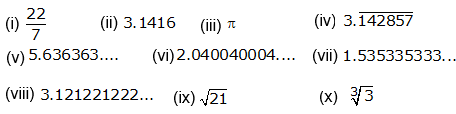Solution:

(i) 22/7 is a rational number.

(ii) 3.1416 is a rational number.

It is a terminating decimal and non-repeating decimal.

(iii) π is an irrational number.

It is a non-terminating and non-repeating decimal.

(iv) $3.\overline{142857}$

A rational number. Non-terminating repeating decimal.

(v) 5.636363…

A rational number. A non-terminating repeating decimal.

(vi) 2.040040004…

An irrational number. It is a non-terminating and non-repeating decimal.

(vii) 1.535335333…

An irrational number. A non-terminating and non-repeating decimal.

(viii) 3.121221222…

An irrational number. A non-terminating and non-repeating decimal.

(ix)$\sqrt{21}$

An irrational number.

21 = 3 × 7 is an irrational number. And 3 and 7 are prime and irrational numbers.

(x)$\sqrt{3}$

An irrational number.

3 is a prime number. So, 3 is an irrational number.

Question 3: Prove that each of the following numbers is irrational.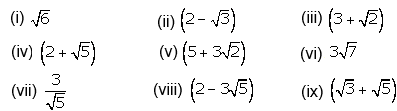Solution:

(i) √6

Let us suppose that √6 is a rational number.

There exists two co-prime numbers, say p and q

So, √6 = p/q

Squaring both sides, we get

6 = p2/q2

or 6q2 = p2 …(1)

Which shows that, p² is divisible by 6

This implies, p is divisible by 6

Let p = 6a for some integer a

Equation (1) implies: 6q² = 36a²

⇨ q² = 6a²

q² is also divisible by 6

⇨ q is divisible by 6

6 is common factors of p and q

But this contradicts the fact that p and q have no common factor

Our assumption is wrong. Thus, √6 is irrational

(ii) (2 – √3)

Let us assume that (2 – √3) is a rational.

Subtract given number form 2, considering 2 is a rational number.

As we know, Difference of two rational numbers is a rational.

So, 2 – (2 – √3 ) is rational

⇨ √3 is rational

Thus, (2 – √3) is an irrational.

(iii) (3 + √2 )

Let us assume that (3 + √2 ) is rational.

Subtract 3 form given number, considering 3 is a rational number.

As we know, Difference of two rational numbers is a rational.

(3 + √2 ) – 3 is rational

⇨ √2 is rational

Which is contradictory to our assumption.

So, (3 + √2 ) is irrational

(iv) (2 + √5 )

Let us assume that (2 + √5 ) is rational.

Subtract 2 form given number, considering 2 is a rational number.

As we know, Difference of two rational numbers is a rational.

(2 + √5) – 2 is rational

⇨ √5 is rational

Which is contradictory to our assumption.

So, (2 + √5) is irrational

(v) (5 + 3√2 )

Let us assume that (5 + 3√2 ) is rational.

Subtract 5 form given number, considering 5 is a rational number.

As we know, Difference of two rational numbers is a rational.

(5 + 3√2 ) – 5 is rational

⇨ 3√2 is rational

And, 3 is rational and √2 is rational.

(Product of two rational numbers is rational)

⇨√2 is rational

Which is contradictory to our assumption.

So, (5 + 3√2 ) is irrational

(vi) 3√7

Let us assume that 3√7 is rational

Here 3 is rational and √7 is rational.

As we know, Product of two rational numbers is rational.

But √7 is rational, which is contradictory to our assumption.

So, 3√7 is irrational

(vii) 3 / √5

Let us assume that 3 / √5 is rational

3 / √5 x √5/√5 = 3√5/5

Where 3√5/5 is rational

Which shows that 3/5 is rational and √5 is rational.

But the fact is √5 is an irrational.

Our assumption is wrong, and

3 / √5 is irrational

(viii)(2 – 3√5)

Let us assume that 2 – 3√5 is rational.

Subtract given number form 2, considering 2 is a rational number.

As we know, Difference of two rational numbers is a rational.

2 – (2 – 3√5) is rational

⇨ 3√5 is rational

Above result is possible if 3 is rational and √5 is rational.

Because, product of two rational numbers is rational

But the fact is √5 is an irrational

Our assumption is wrong, and

(2 – 3√5) is irrational

(ix) (√3 + √5)

Let us assume that √3 + √5 is rational

On squaring, we get

(√3 + √5)² is rational

⇨ 3 + 2√3 x √5 + 5 is rational

⇨ 8 + 2√15 is rational

Subtract 8 from above result, considering 8 is a rational number.

As we know, Difference of two rational numbers is a rational.

⇨ 8 + 2√15 – 8 is rational

⇨ 2√15 is rational

Which is only possible if 2 is rational and √15 is rational.

The fact is √15 is not a rational number.

Our assumption is wrong, and

(√3 + √5) is irrational.

Question 4. Prove that 1/√3 is irrational.

Solution:

Let us assume that 1/√3 is rational

⇨ 1/√3 x √3/ √3 = √3/3 is rational

Which is only possible if 1/3 is rational and √3 is rational. As we know that, Product of two rational numbers is rational

But the fact is, √3 is an irrational.

Which is contradictory to our assumption.

which implies 1/√3 is irrational. Hence proved.

Question 5: (i) Give an example of two irrationals whose sum is rational.

(ii) Give an example of two irrationals whose product is rational.

Solution:

(i) Let us consider two numbers 2 + √3 and 2 – √3 which are irrationals

their sum = (2 + √3) + (2 – √3) = 4; Which is a rational numbers.

(ii) Let us consider two numbers 3 + √2 and 3 – √2 which are irrationals.

their product = (3 + √2) (3 – √2)

= (3)² – (√2)²

= 9 – 2 = 7; which is a rational number.

Question 6.

State whether the given statement is true or false.

(i) The sum of two rationals is always rational.

(ii) The product of two rationals is always rational.

(iii) The sum of two irrationals is always an irrational.

(iv) The product of two irrationals is always an irrational.

(v) The sum of a rational and an irrational is irrational.

(vi) The product of a rational and an irrational is irrational.

Solution:

(i) True.

(ii) True.

(iii) False.

Reason:

Sum of two irrational can be rational. Let us take an example,

Consider two irrational numbers: (3 + √2) and (3 – √2)

Sum: (3 + √2) + (3 – √2) = 3 + √2 + 3 – √2 = 6 which is rational.

(iv) False.

Reason:

Product of two irrational numbers can be rational. Let us take an example,

Consider two irrational numbers: (3 + √2) and (3 – √2)

Product: (3 + √2)(3 – √2 ) = (3)2 – (√2 )2 = 9 – 2 = 7; which is rational

(v) True.

(vi) True.

Question 7. Prove that (2√3 – 1) is an irrational number.

Solution:

Let us assume that (2√3 – 1) is a rational.

Add 1 to the above number, considering 1 is a rational number.

As we know, sum of two rational numbers is a rational.

Sum = 2√3 – 1 + 1 = 2√3

Which is only possible if 2 is rational and √3 is rational.

As we know that product of two rational numbers is rational.

But the fact is √3 is an irrational number which contradicts to our assumption.

So, (2√3 – 1) is an irrational.

Question 8. Prove that (4 – 5√2 ) is an irrational number.

Solution:

Let us assume that (4 – 5√2 ) is a rational.

Subtract given number form 4, considering 4 is a rational number.

As we know, Difference of two rational numbers is a rational.

4 – (4 – 5√2 ) is rational

⇨ 5√2 is rational

Which is only possible if 5 is rational and √2 is rational

As we know, product of two rational number is rational.

But the fact is √2 is an irrational.

Which is contradict to our assumption.

Hence, 4 – 5√2 is irrational. Hence Proved.

Question 9. Prove that (5 – 2√3) is an irrational number.

Solution:

Let us assume that (5 – 2√3) is a rational.

Subtract given number form 5, considering 5 is a rational number.

As we know, Difference of two rational numbers is a rational.

⇨ 5 – (5 – 2√3) is rational

⇨ 2√3 is rational

Which is only possible if 2 is rational and √3 is rational

As we know, product of two rational number is rational.

But the fact is √3 is an irrational.

Which is contradict to our assumption.

(5 – 2√3) is an irrational number.

Question 10: Prove that 5√2 is irrational.

Solution:

Let us assume that 5√2 is a rational.

Which is only possible if 5 is rational and √2 is rational

As we know, product of two rational number is rational.

But the fact is √2 is an irrational.

Which is contradict to our assumption.

5√2 is an irrational. Hence proved.

Question 11: Prove that 2/√7 is irrational.

Solution:

Let us assume that 2/√7 is a rational number.

2/√7 x √7/√7 = 2√7/7 is a rational number

Which is only possible if 2/7 is rational and √7 is rational.

But the fact is √7 is an irrational.

Which is contradicts to our assumption.

2/√7 is an irrational number. Hence proved.

## RS Aggarwal Solutions for Class 10 Maths Chapter 1 Real Numbers Exercise 1D

Class 10 Maths Chapter 1 Real Numbers Exercise 1D, based on the following topics and subtopics:

• Irrational Numbers
• Important results on Irrational